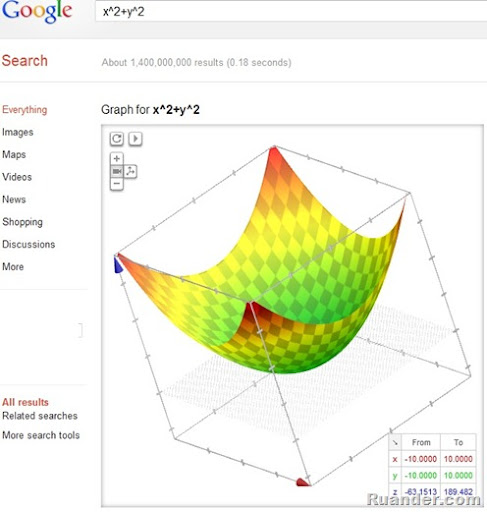## Pages

### Plot Mathematical Expressions Using Google

Need a quick and easy way to plot and visualize mathematical expressions? Currently, there are several options for 2D expressions, but how about 3D? Well, if you are using the latest version of Chrome or Firefox, then it is just a matter of typing the equation on Google Search and you will have your plot in less than a second. Currently, you can plot explicit 2D and 3D expressions.  You can also control the x, y, and z limits of your plot. Below is an example for the expression z=x^2+y^2. Give it a try and see what you think.